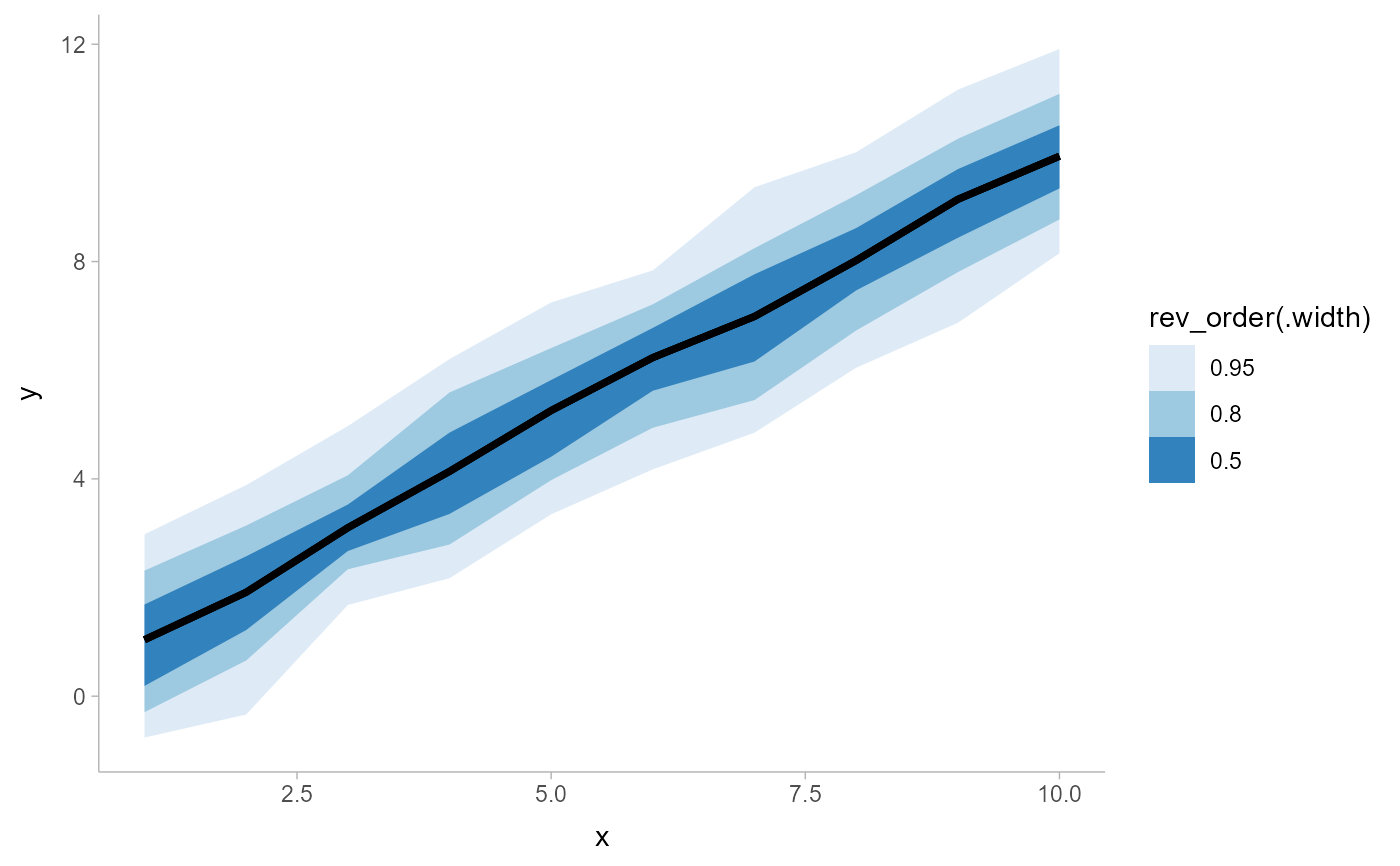A combination of geom_line() and geom_ribbon() with default aesthetics designed for use with output from point_interval().

geom_lineribbon(
mapping = NULL,
data = NULL,
stat = "identity",
position = "identity",
...,
step = FALSE,
orientation = NA,
na.rm = FALSE,
show.legend = NA,
inherit.aes = TRUE
)

## Arguments

mapping Set of aesthetic mappings created by aes() or aes_(). If specified and inherit.aes = TRUE (the default), it is combined with the default mapping at the top level of the plot. You must supply mapping if there is no plot mapping. The data to be displayed in this layer. There are three options: If NULL, the default, the data is inherited from the plot data as specified in the call to ggplot(). A data.frame, or other object, will override the plot data. All objects will be fortified to produce a data frame. See fortify() for which variables will be created. A function will be called with a single argument, the plot data. The return value must be a data.frame, and will be used as the layer data. A function can be created from a formula (e.g. ~ head(.x, 10)). The statistical transformation to use on the data for this layer, as a string. Position adjustment, either as a string, or the result of a call to a position adjustment function. Other arguments passed to layer(). Should the line/ribbon be drawn as a step function? One of: FALSE (do not draw as a step function, the default), TRUE (draw a step function using the "mid" approach), "mid" (draw steps midway between adjacent x values), "hv" (draw horizontal-then-vertical steps), "vh" (draw as vertical-then-horizontal steps). TRUE is an alias for "mid" because for a step function with ribbons, "mid" is probably what you want (for the other two step approaches the ribbons at either the vert first or vert last x value will not be visible). Whether this geom is drawn horizontally ("horizontal") or vertically ("vertical"). The default, NA, automatically detects the orientation based on how the aesthetics are assigned, and should generally do an okay job at this. When horizontal (resp. vertical), the geom uses the y (resp. x) aesthetic to identify different groups, then for each group uses the x (resp. y) aesthetic and the thickness aesthetic to draw a function as an slab, and draws points and intervals horizontally (resp. vertically) using the xmin, x, and xmax (resp. ymin, y, and ymax) aesthetics. For compatibility with the base ggplot naming scheme for orientation, "x" can be used as an alias for "vertical" and "y" as an alias for "horizontal" (tidybayes had an orientation parameter before ggplot did, and I think the tidybayes naming scheme is more intuitive: "x" and "y" are not orientations and their mapping to orientations is, in my opinion, backwards; but the base ggplot naming scheme is allowed for compatibility). If FALSE, the default, missing values are removed with a warning. If TRUE, missing values are silently removed. logical. Should this layer be included in the legends? NA, the default, includes if any aesthetics are mapped. FALSE never includes, and TRUE always includes. It can also be a named logical vector to finely select the aesthetics to display. If FALSE, overrides the default aesthetics, rather than combining with them. This is most useful for helper functions that define both data and aesthetics and shouldn't inherit behaviour from the default plot specification, e.g. borders().

## Value

A ggplot2::Geom representing a combined line+uncertainty ribbon geometry which can be added to a ggplot() object.

## Details

geom_lineribbon is a combination version of a geom_line(), and geom_ribbon designed for use with output from point_interval(). This geom sets some default aesthetics equal to the .width column generated by the point_interval family of functions, making them often more convenient than a vanilla geom_ribbon() + geom_line().

Specifically, geom_lineribbon acts as if its default aesthetics are aes(fill = forcats::fct_rev(ordered(.width))).

See stat_lineribbon() for a version that does summarizing of samples into points and intervals within ggplot. See geom_pointinterval() for a similar geom intended for point summaries and intervals. See geom_ribbon() and geom_line() for the geoms this is based on.

Matthew Kay

## Examples


library(dplyr)
library(ggplot2)

theme_set(theme_ggdist())

tibble(x = 1:10) %>%
group_by_all() %>%
do(tibble(y = rnorm(100, .\$x))) %>%
median_qi(.width = c(.5, .8, .95)) %>%
ggplot(aes(x = x, y = y, ymin = .lower, ymax = .upper)) +
# automatically uses aes(fill = forcats::fct_rev(ordered(.width)))
geom_lineribbon() +
scale_fill_brewer()